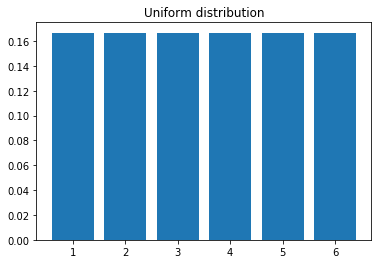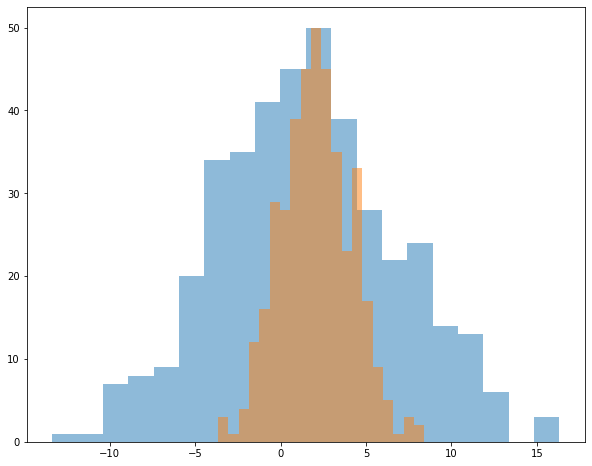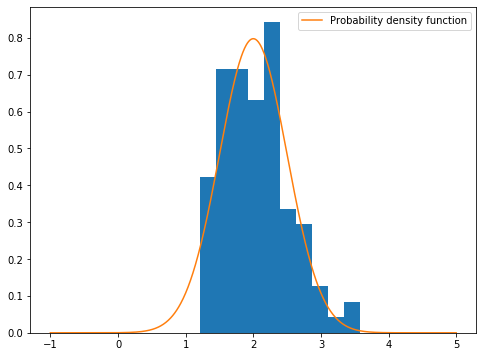# Mlf-Statistical Techniques

Last updated: September 7th, 2020# Statistical techniques¶

Statistics is a field of mathematics that is universally agreed to be a prerequisite for a deeper understanding of machine learning. We will review basic statistics concepts necessary to understand machine learning and apply them using pandas and numpy.

Overview:

• Introduction to statistics
• Descriptive Statistics using Pandas and numpy
• Measure of central tendency and dispersions
• Visualization of statistics data.## Introduction¶

Statistics are mainly classified into two sub-branches:

• Descriptive statistics: These are used to summarize data, such as the mean, standard deviation for continuous data types (such as age), whereas frequency and percentage are useful for categorical data (such as gender).

• Inferential statistics: Many times, collecting the entire data (also known as population in statistical methodology) is impossible, hence a subset of the data points is collected, also called a sample, and conclusions about the entire population will be drawn, which is known as inferential statistics. Inferences are drawn using hypothesis testing, the estimation of numerical characteristics, the correlation of relationships within data, and so on.## Statistical terminology¶

We will review important concepts:

• Population: This is the totality, the complete list of observations, or all the data points about the subject under study.

• Sample: A sample is a subset of a population, usually a small portion of the population that is being analyzed.• Parameter versus statistic: Any measure that is calculated on the population is a parameter, whereas on a sample it is called a statistic.## Types of Descriptive Statistics?¶

Descriptive statistics are broken down into two categories. Measures of central tendency and measures of variability (spread).

#### Measure of Central Tendency¶

Central tendency refers to the idea that there is one number that best summarizes the entire set of measurements, a number that is in some way central to the set.

Mean: This is a simple arithmetic average, which is computed by taking the aggregated sum of values divided by a count of those values. The mean is sensitive to outliers in the data. An outlier is the value of a set or column that is highly deviant from the many other values in the same data; it usually has very high or low values.

Median: This is the midpoint of the data, and is calculated by either arranging it in ascending or descending order. If there are N observations.

Mode: This is the most repetitive data point in the dataThe Python code for the calculation of mean, median, and mode using a numpy array and the stats package is as follows:

In :
import numpy as np
from scipy import stats

data = np.array([4,5,1,2,7,2,6,9,3])

# Calculate Mean
dt_mean = np.mean(data) ; print ("Mean :",round(dt_mean,2))

# Calculate Median
dt_median = np.median(data) ; print ("Median :",dt_median)

# Calculate Mode
dt_mode = stats.mode(data); print ("Mode :",dt_mode)

Mean : 4.33
Median : 4.0
Mode : 2


Now we review how to calculate mean, median and mode with pandas using IRIS dataset.

This is perhaps the best known database to be found in the machine learning literature. Fisher's paper is a classic in the field and is referenced frequently to this day. The data set contains 3 classes of 50 instances each, where each class refers to a type of iris plant. One class is linearly separable from the other 2; the latter are NOT linearly separable from each other.

Attribute Information:

1. Sepal length in cm
2. Sepal width in cm
3. Petal length in cm
4. Petal width in cm
5. Class:
-- Iris Setosa
-- Iris Versicolour
-- Iris Virginica

Information about the original paper and usages of the dataset can be found in the UCI Machine Learning Repository -- Iris Data Set.

Source

###### Fisher,R.A. "The use of multiple measurements in taxonomic problems" Annual Eugenics, 7, Part II, 179-188 (1936); also in "Contributions to Mathematical Statistics" (John Wiley, NY, 1950).¶
In :
import seaborn as sns
import pandas as pd

iris = sns.load_dataset('iris')
iris.head()

Out:
sepal_length sepal_width petal_length petal_width species
0 5.1 3.5 1.4 0.2 setosa
1 4.9 3.0 1.4 0.2 setosa
2 4.7 3.2 1.3 0.2 setosa
3 4.6 3.1 1.5 0.2 setosa
4 5.0 3.6 1.4 0.2 setosa
In :
df_mean=iris['sepal_length'].mean(); print ("Mean :",round(df_mean,2))
df_median=iris['sepal_length'].median(); print ("Median :",round(df_median,2))
df_mode=iris['sepal_length'].mode(); print ("Mode :",round(df_mode,2))

Mean : 5.84
Median : 5.8
Mode : 5.0#### Measure of variability:¶

The Dispersion is the variation in the data, and measures the inconsistencies in the value of variables in the data. Dispersion actually provides an idea about the spread rather than central values.

• Range: This is the difference between the maximum and minimum of the value.
• Variance: This is the mean of squared deviations from the mean ( N = number of data points). The dimension of variance is the square of the actual values. The reason to use denominator N-1 for a sample instead of N in the population is due the degree of freedom. 1 degree of freedom lost in a sample by the time of calculating variance is due to extraction of substitution of sample:

• Standard deviation: This is the square root of variance. By applying the square root on variance, we measure the dispersion with respect to the original variable rather than square of the dimension.

The table below summarizes the important symbols and formulas for populations and samples, where xi = data points, μ = mean of the data, N = number of data points.• Quantiles: These are simply identical fragments of the data. Quantiles cover percentiles, deciles, quartiles, and so on. These measures are calculated after arranging the data in ascending order:

• Quartile: This is one-fourth of the data, and also is the 25th percentile. The first quartile is 25 percent of the data, the second quartile is 50 percent of the data, the third quartile is 75 percent of the data. The second quartile is also known as the median or 50th percentile or 5th decile.
• Interquartile range: This is the difference between the third quartile and first quartile. It is effective in identifying outliers in data. The interquartile range describes the middle 50 percent of the data points.Source

The python code is as follows:

In :
from statistics import variance, stdev

game_points = np.array([35,56,43,59,63,79,35,41,64,43,93,60,77,24,82])

# Calculate Variance
dt_var = variance(game_points) ; print ("Sample variance:",
round(dt_var,2))
# Calculate Standard Deviation
dt_std = stdev(game_points) ; print ("Sample std.dev:",
round(dt_std,2))
# Calculate Range
dt_rng = np.max(game_points,axis=0) - np.min(game_points,axis=0) ; print ("Range:",dt_rng)
#Calculate percentiles
print ("Quantiles:")
for val in [20,80,100]:
dt_qntls = np.percentile(game_points,val)
print (str(val)+"%" ,round(dt_qntls,2))
# Calculate IQR
q75, q25 = np.percentile(game_points, [75 ,25]); print ("Interquartile range:",q75-q25)

Sample variance: 400
Sample std.dev: 20.0
Range: 69
Quantiles:
20% 39.8
80% 77.4
100% 93.0
Interquartile range: 28.5


Let´s try with pandas sing IRIS dataset

In :
df_std=iris['sepal_length'].std(); print ("std.dev :",round(df_std,2))
df_var=iris['sepal_length'].var(); print ("Variance :",round(df_median,2))

std.dev : 0.83
Variance : 5.8## Probability distribution¶

The probability distribution is a central concept in probability and statistics and therefore there is a lot to be said.Let's start with an example: a six-sided dice. The probability that when rolling the dice one face is $1/6$. If we graph the probability for each possible result of rolling a died, we would obtain the following graph:

In :
import matplotlib.pyplot as plt

val = np.arange(1,7)
test = np.zeros(6) + 1/6
plt.bar(val, test)
plt.title('Uniform distribution')
plt.show()In this case, we said that the probability distribution is uniform, since it assigns the same probability to each value that can come out when rolling the dice.

Technical details:

1. The result of rolling a died is an example of a random variable.
2. In this case a random variable can take discrete and bounded (limited) values: 1, 2, 3, 4, 5 and 6
3. There are random variables where the possible values it can take are continuous and unbounded. We will see the most famous distribution of them below.

### Normal Distribution¶

In probability theory, a normal (or Gaussian or Gauss or Laplace–Gauss) distribution is a type of continuous probability distribution for a real-valued random variable. Normal distributions are important in statistics and are often used in the natural and social sciences to represent real-valued random variables whose distributions are not known.

Source

We will use numpy to generate a random normal distribution

In :
average = 2.0
std_1 = 5.0
std_2 = 2.0
s_1 = np.random.normal(loc = average, scale = std_1, size = 400)
s_2 = np.random.normal(loc = average, scale = std_2, size = 400)
plt.figure(figsize = (10,8))
plt.hist(s_1, bins = 20, alpha = 0.5)
plt.hist(s_2, bins = 20, alpha = 0.5)
plt.show()### Exercise¶

Generate 100 samples of a normal distribution of mean $\ mu$ and standard deviation $\ sigma$ that take the following values:

• $\ mu = 2$, $\ sigma = 0.5$
• $\ mu = 8$, $\ sigma = 10$

1 - What is the mean value of the samples obtained? Does it match $\ mu$?

2 - Plot the histogram of the samples obtained, making a figure for each case. Do you dare to superimpose the theoretical distribution on the graph? use scipy for the last question.

In :
import seaborn as sns
from scipy import stats

mu = 2
sigma = 0.5
s = np.random.normal(loc = mu, scale = sigma, size = 100)
print(s.mean())

x = np.linspace(-1,5, 1000)
y = stats.norm.pdf(x, mu, sigma)

plt.figure(figsize = (8,6))
plt.hist(s,normed=True)
plt.plot(x,y, label = 'Probability density function')
plt.legend()
plt.show()

2.046445831746809

<ipython-input-8-02d702ef535f>:13: MatplotlibDeprecationWarning:
The 'normed' kwarg was deprecated in Matplotlib 2.1 and will be removed in 3.1. Use 'density' instead.
plt.hist(s,normed=True)